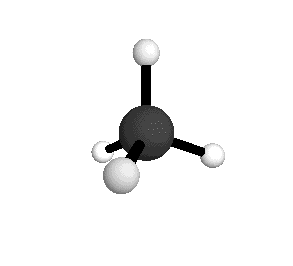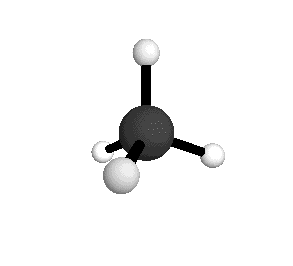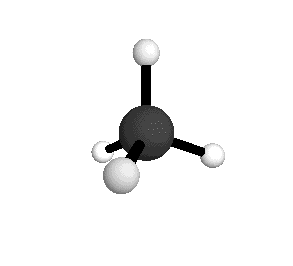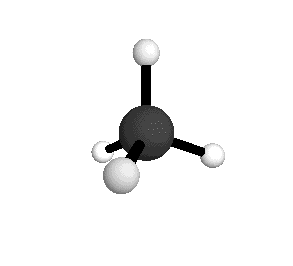# How do we know that methane is tetrahedral?

##### 1 Answer
Jan 13, 2015

Even though we can't see the molecules, we can tell much about them from the physical and chemical properties of the compound.

For example, we know by chemical analysis that the molecular formula is CH₄.

We know that all the H atoms are equivalent.

If we replace one of them with a Cl atom, we get only one form of CH₃Cl.

If there were different types of hydrogens, we would get different forms of CH₃Cl, depending on which H atom was replaced.

There are only two possible structures that have equivalent hydrogens.

Square planarTetrahedralWe know that square planar is wrong because, if we substitute two of the H atoms with Cl, we would get two different forms of CH₂Cl₂.

One would have the two C-Cl bonds at 90° to each other, and the other would have the bonds at 180° to each other. There is only one form of CH₂Cl₂.The tetrahedral structure correctly predicts the existence of only one molecule with the formula CH₂Cl₂.

From the tetrahedral structure, we can calculate the H-C-H bond angles to be 109° 28'.

Methane molecules are constantly rotating and vibrating. The energies of these motions are quantized.From the spectra we can calculate very precise values of molecular bond lengths and angles.

And that's how we know the structure of a methane molecule even though we can't see it.